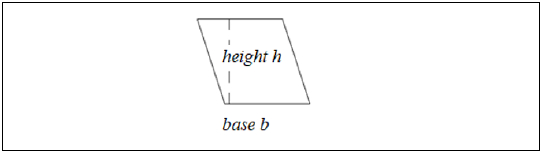# Java Program to Find the Area of a Parallelogram

In this article, we will understand how to find the area of a parallelogramA parallelogram has two pairs of parallel equal opposite sides. It has a base and a height which is the perpendicular distance between the base and its opposite parallel side.

The area of a parallelogram is calculated using the formula −

base * height
i.e.
b x h

Below is a demonstration of the same −Input

Suppose our input is −

Base : 6
Height : 8

Output

The desired output would be −

Area parallelogram is : 48


## Algorithm

Step 1 - START
Step 2 - Declare three integer values values namely
Step 3 - Read the required values from the user/ define the values
Step 4 - Calculate the area using the formula by base * height and store the result.
Step 5 - Display the result
Step 6 - Stop

## Example 1

Here, the input is being entered by the user based on a prompt. You can try this example live in ourcoding ground tool.

import java.util.Scanner;
public class AreaOfParallelogram{
public static void main(String args[]){
int base, height, my_area;
System.out.println("Required packages have been imported");
Scanner my_scanner = new Scanner(System.in);
System.out.println("A reader object has been defined ");
System.out.print("Enter the value of parallelogram base : ");
base = my_scanner.nextInt();
System.out.print("Enter the value of parallelogram base : ");
height = my_scanner.nextInt();
my_area=base*height;
System.out.println("The area of the parallelogram is : "+my_area);
}
}

## Output

Required packages have been imported
A reader object has been defined
Enter the value of parallelogram base : 6
Enter the value of parallelogram base : 8
The area of the parallelogram is : 48

## Example 2

Here, the integer has been previously defined, and its value is accessed and displayed on the console.

public class AreaOfParallelogram{
public static void main(String args[]){
int base, height;
base=6;
height=8;
System.out.println("The base and height values are defined as " +base +" and " +height);
int my_area=base*height;
System.out.println("The area of the parallelogram is : "+my_area);
}
}

## Output

The base and height values are defined as 6 and 8
The area of the parallelogram is : 48